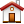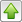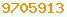pi/π : Java Glossary

pi/π
An irrational number approximately 3.141592654, the ratio of the circumference of a circle to its diameter. It is approximated in Java with the double Math.PI.

The circumference of a circle is 2πr where r is the radius.

The area of a circle is πr² where r is the radius.

A complete circle is radians. One radian is about 57.2957795 degrees, measured counterclockwise from due east. Java’s Math.sin and Math.cos etc. work in radians.

45 degrees = π/4 = .775398 radians.
90 degrees = π/2 = 1.57079 radians.
180 degrees = π = 3.14159 radians.
360 degrees = = 6.28318 radians.

The circumference of the outer curved part of a pie-shaped wedge is θr where θ is the angle in radians and r is the radius.

The area of a pie-shaped wedge is θr²/2 where θ is the angle in radians and r is the radius.

There are two convenience methods for interconverting degress and radians.

// built-in radians <-> degrees methods.
public static double toDegrees ( double angleInRadians );
public static double toRadians ( double angleInDegrees );This page is postedon the web at: http://mindprod.com/jgloss/pi.html Optional Replicator mirror of mindprod.com on local hard disk J: J:\mindprod\jgloss\pi.htmlPlease read the feedback from other visitors, or send your own feedback about the site.Contact Roedy. Please feel free to link to this page without explicit permission. Canadian Mind Products IP:[65.110.21.43] Your face IP:[18.204.227.250] Feedback You are visitor number